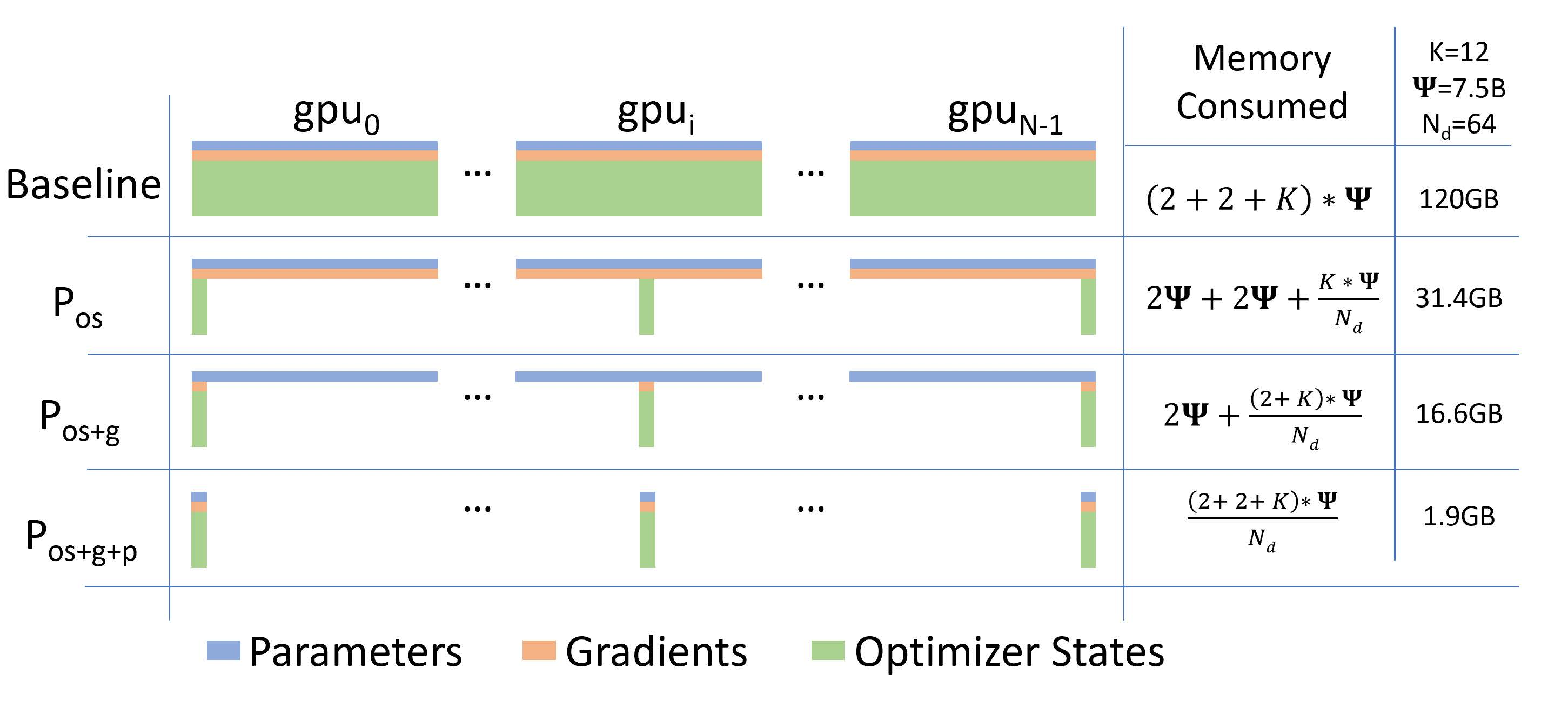# Zero Redundancy Optimizer (ZeRO)¶

## ZeRO 简介¶

Zero Redundancy Optimizer (ZeRO) 是论文 ZeRO: Memory Optimization Towards Training A Trillion Parameter Models 提出的一种用于减少数据并行策略下的显存占用的方法。

2. 残余状态(residual states)。包括激活函数、临时缓冲区和不可用的内存碎片。

ZeRO-DP 可以分为三个阶段，通过对 OPG 状态进行分区而不是直接复制来消除内存冗余，每个 GPU 仅保存部分 OPG。具体来说，ZeRO-DP 有三个主要的优化阶段，分别对应 O、P 和 G。三个阶段逐级递加：

• 阶段1，优化器状态分区（Pos）：显存消耗减少 4 倍，通信量与数据并行相同。
• 阶段2，添加梯度分区优化（Pos+g）：显存消耗减少 8 倍，通信量与数据并行相同。
• 阶段3，添加参数分区优化（Pos+g+p）：模型占用的显存被平均分配到每个 GPU 中，显存消耗量与数据并行的并行度成线性反比关系，但通信量会有些许增加。## ZeRO 使用示例¶

import oneflow as flow
from oneflow import nn


### 定义数据并行训练流程¶

Note

P = flow.placement("cuda", ranks=[0, 1])
S0 = flow.sbp.split(0)
DEVICE = "cuda"


model = nn.Sequential(nn.Linear(256, 128),
nn.ReLU(),
nn.Linear(128, 10))
model = model.to(DEVICE)
model.train()
model = model.to_global(placement=P, sbp=B)

loss_fn = nn.CrossEntropyLoss().to(DEVICE)
optimizer = flow.optim.SGD(model.parameters(), lr=1e-3)


ZeRO 是在 nn.Graph 的图编译器中实现的，因此需要将动态图模型转换为 nn.Graph：

class CustomGraph(flow.nn.Graph):
def __init__(self):
super().__init__()
self.model = model
self.loss_fn = loss_fn

# TODO: 设置 ZeRO

def build(self, x, y):
preds = self.model(x)
loss = self.loss_fn(preds, y)
loss.backward()
return preds


graph_model = CustomGraph()

for _ in range(100):
x = flow.randn(128, 256).to(DEVICE)
y = flow.ones(128, 1, dtype=flow.int64).to(DEVICE)
global_x = x.to_global(placement=P, sbp=S0)
global_y = y.to_global(placement=P, sbp=S0)

graph_model(global_x, global_y)


### 在 nn.Graph 中开启 ZeRO¶

#### 开启阶段1优化¶

class CustomGraph(flow.nn.Graph):
def __init__(self):
super().__init__()
...
# 设置 ZeRO 开启 stage 1
self.config.enable_zero(True, stage=1)
...


Note

#### 开启阶段 2 优化¶

class CustomGraph(flow.nn.Graph):
def __init__(self):
super().__init__()
...
# 设置 ZeRO 开启 stage 2
self.config.enable_zero(True, stage=2)
...


class CustomGraph(flow.nn.Graph):
def __init__(self):
super().__init__()
...
# 设置 ZeRO 开启 stage 2
self.config.enable_zero()
...


#### 开启阶段 3 优化¶

class CustomGraph(flow.nn.Graph):
def __init__(self):
super().__init__()
...
# 设置 ZeRO 开启 stage 3
self.config.enable_zero(True, stage=3)
...